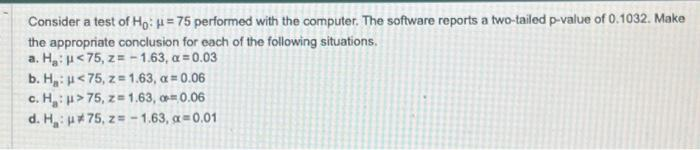Home / Expert Answers / Statistics and Probability / consider-a-test-of-ho-75-performed-with-the-computer-the-software-reports-a-two-tailed-p-value-pa384

# (Solved): Consider a test of Ho: =75 performed with the computer. The software reports a two-tailed p-value ...

Consider a test of Ho: μ=75 performed with the computer. The software reports a two-tailed p-value of 0.1032. Make the appropriate conclusion for each of the following situations. a. H₂: μ<75, z = -1.63, α = 0.03 b. H₂: μ< 75, z = 1.63, α = 0.06 c. H₂ μ>75, z= 1.63, α=0.06 d. H₂ μ not = 75, z = -1.63, α=0.01Consider a test of performed with the computer. The software reports a two-tailed p-value of 0.1032 . Make the appropriate conclusion for each of the following situations. a. b. c. d.

We have an Answer from Expert

(a)
H0 :   = 75
Ha :   < 75 (left tailed)

The test statistic, z = -1.63

p-value = P(Z<-1.63) = 0.1032/2 = 0.0516

= 0.03
We have an Answer from Expert